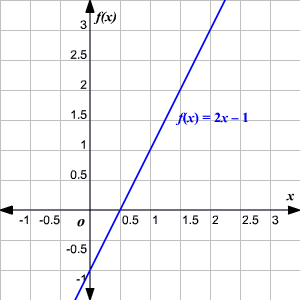# Linear Function

A linear function is a function that can be written in the form $f\left(x\right)=mx+b$ where $m$ and $b$ are real numbers and $m\ne 0$ .

Example:

Graph the function $f\left(x\right)=2x-1$ .The graph is always a line with either positive or negative slope .